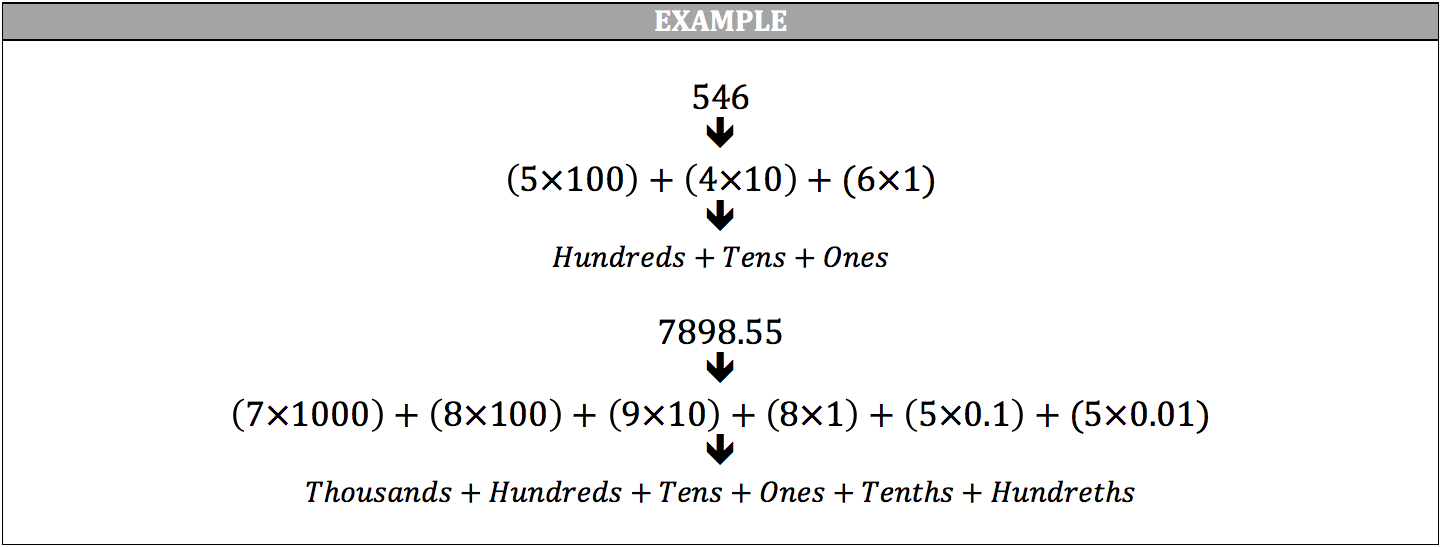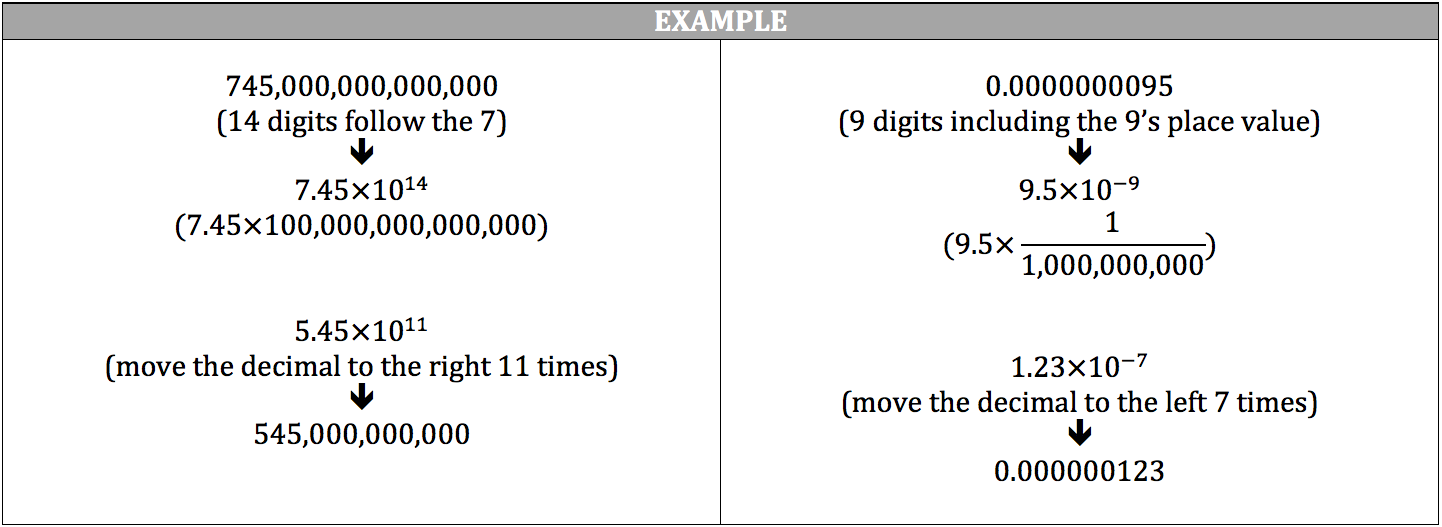A basic representation of a number, such as 546, is designated as standard notation. Expanded notation demonstrates the place value for each digit in a number by multiplying each digit by its place value and adding all the digits together. You do not have to multiply 1 to the one’s place, but it can help with remembering what place value you are on when working with numbers with many place values.For incredibly large or small numbers, such as 745,000,000,000,000 or 0.0000000095, scientific notation is used to show these numbers in more manageable forms. Scientific notation converts the original number into a number with a one’s place value followed by a few decimal place values. This new number is then multiplied by 10 taken to a certain exponent and left in this form.

For numbers above the one’s place value, move the decimal to the left until the largest place value is converted to the one’s place value. The number of times you moved the decimal to the left is the 10’s power and it will be positive. For numbers below the one’s place value, move the decimal to the right until the first non‐zero digit is converted to the one’s place value. The number of times you moved the decimal to the right is the 10’s power and it will be negative.